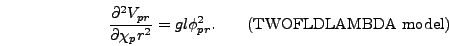Next: Writing a Model File Up: Writing Down the Equations Previous: The Potential Derivative

### Effective Masses

Finally, for setting the initial field fluctuations and later for calculating spectra of number density and energy density the program needs formulas for the effective masses of the fields. In program variables, these are given by (see section 6.3.2)(4.14)

so you will need to calculate these second derivatives. For the TWOFLDLAMBDA model(4.15)(4.16)